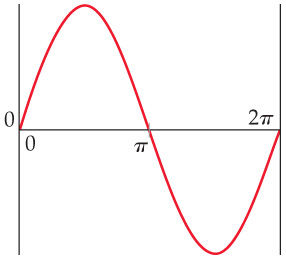# Problem: Consider a fictitious one-dimensional system with one electron. The wave function for the electron, drawn below, is Ψ(x) = sin x from x = 0 to x = 2π..What is the probability that the electron will be found at x = π? What is such a point in a wave function called?

###### FREE Expert Solution
80% (483 ratings)
###### Problem Details

Consider a fictitious one-dimensional system with one electron. The wave function for the electron, drawn below, is Ψ(x) = sin x from x = 0 to x = 2π..What is the probability that the electron will be found at x = π? What is such a point in a wave function called?